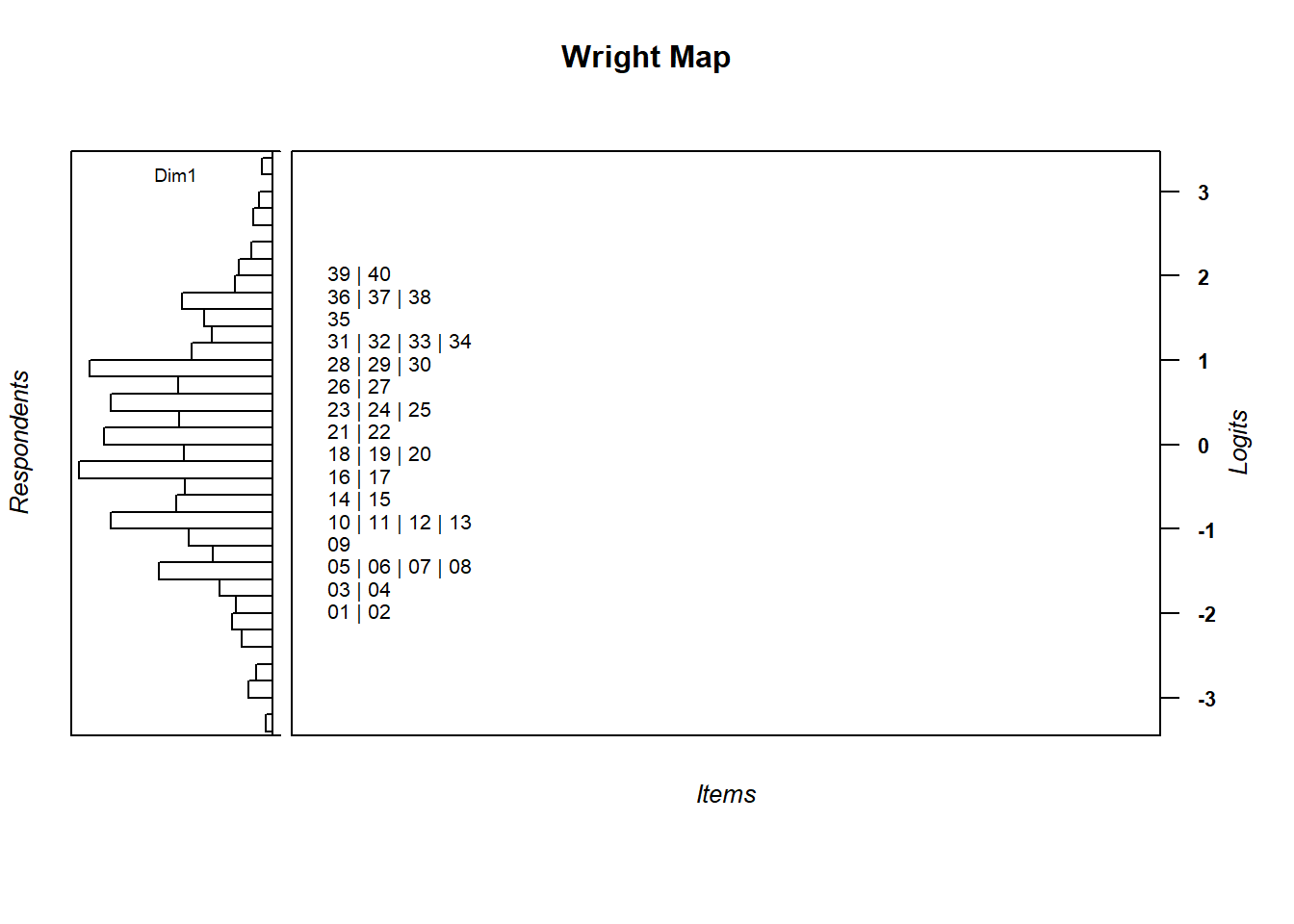# Chapter 4 Fitting the Rasch model

This session will focus on hands-on practice of using R for estimating the Rasch model.

## 4.1 Exercise 1

Analyse the data set data.sim.rasch in the TAM package using the following R code:

rm(list=ls())  #remove all variables in the R environment

library(TAM)  #load the package TAM so we can use the functions in TAM

#First, analyse the data set, data.sim.rasch in the TAM package.
data("data.sim.rasch")  #call up the data set so we can use it
head(data.sim.rasch)  #see what's in the data set

#run a joint maximum likelihood estimation of the Rasch model
mod1 <- tam.jml(data.sim.rasch)

#All the results of the Rasch analysis are stored in the object called "mod1"
summary(mod1)  #see a summary of the results

#See specific results from the Rasch analysis
mod1$item mod1$WLE
mod1$WLEreliability #You can calculate item and person summary statistics summary(mod1$item1)
summary(mod1$WLE) hist(mod1$WLE)

#You can plot ICCs
plot(mod1)

If you have problems exporting the ICCs to files, you can try a couple of ways.

First, you can put them in a pdf document, such as

pdf("ICCplots.pdf")
plot(mod1)
dev.off()

Note that sometimes I have to run dev.off() twice, otherwise all output still goes into a pdf file. Sometimes I have to run the command dev.new(RStudioGD) to make sure graphics appear in RStudio again. Use dev.cur() to see which graphical device is currently in use. I am quite confused about R graphic devices, obviously!!

If exporting ICCs does not work for you, you can export them individually by running the code:

for (i in 1:mod1$nitems){ plot(mod1,items=i) }  The ICCs plotted have two curves in each graph. The smooth blue curve shows the theoretical ICC according to the Rasch probability (3.1). The black lines joined at six points show the observed ICC from the data. Since the data file data.sim.rasch is simulated according to the Rasch model, the observed curve closely match the theoretical curve. ## 4.2 Plot Wright Map A very useful plot is the Wright map, which plots the estimated ability distribution and the item locations on one graph. This map is also called an Item-person map. We will use the WrightMap package to do this. Make sure you have installed the WrightMap package. library(WrightMap) wrightMap(mod1$WLE, mod1\$xsi, item.side = itemClassic)

The Wright Map is shown in Figure 4.1.Figure 4.1: Wright Map

On the left-side of Figure 4.1, the student ability distribution is shown. On the right-side, the locations of items are shown, as indicated by the item numbers. As the simulated data set had increasing item difficulties from Item 1 to Item 40, the Wright map shows that Items 1 and 2 are the easiest, and Items 39 and 40 are the most difficult. Similarly, students placed at the bottom of the map are of lower abilities, while high ability students are placed on the top of the map. Again, note that item difficulties are defined on the ability scale, so that we can make directly statements about students regarding their chances of successfully answering an item. Under CTT, such a map cannot be constructed since item difficulties and student ability measures are not directly comparable.

## 4.3 Exercise 2

Use the data set, CTTdata, provided in the CTT package to carry out an IRT analysis. Remember to score the data, and use the scored data when calling the tam.jml function.

1. Compare IRT estimated item difficulties with CTT item scores.

Use the R function cor to calculate correlation. Use plot to show the relationship graphically.

2. Compare IRT reliability and CTT reliability

3. Visually compare ‘steepness’ of IRT observed ICC with CTT point-biserial correlation.

4. Compare students’ IRT ability measures (WLE) with students’ test scores (CTT).

Compute correlation and plot the two variables to show the relationship.

## 4.4 Homework

Analyse the data set, data.numeracy, provided by the TAM package. You can take a look at the actual test questions at this link.

1. Which items are most difficult and which are easiest?
2. Compare with CTT results as for Exercise 2 (4.3).

### References

Irribarra, David Torres, and Rebecca Freund. 2014. Wright Map: IRT Item-Person Map with ConQuest Integration. http://github.com/david-ti/wrightmap.